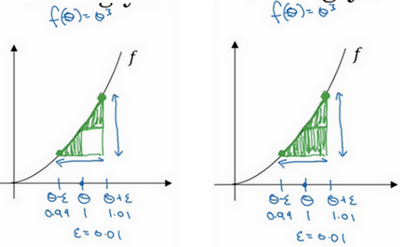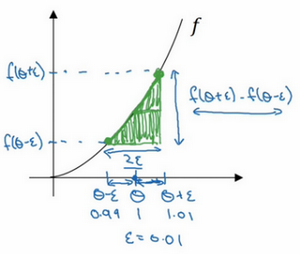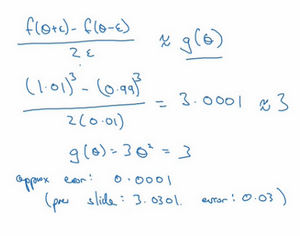# 第二门课 改善深层神经网络：超参数调试、正则化以及优化(Improving Deep Neural Networks:Hyperparameter tuning, Regularization and Optimization)

## 第一周：深度学习的实践层面(Practical aspects of Deep Learning)

### 1.1 训练，验证，测试集（Train / Dev / Test sets）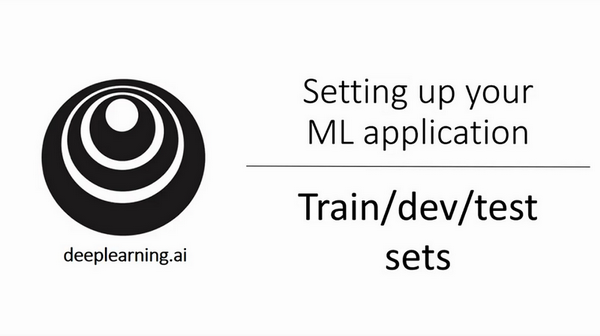1. 神经网络分多少层
2. 每层含有多少个隐藏单元
3. 学习速率是多少
4. 各层采用哪些激活函数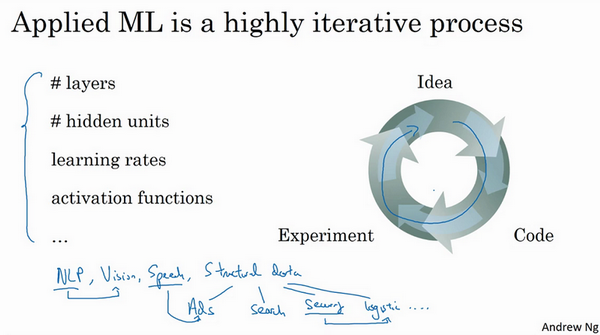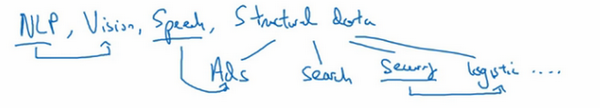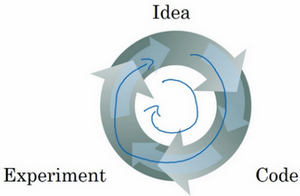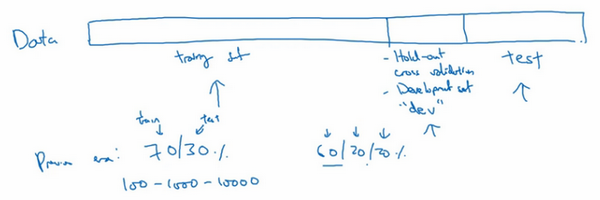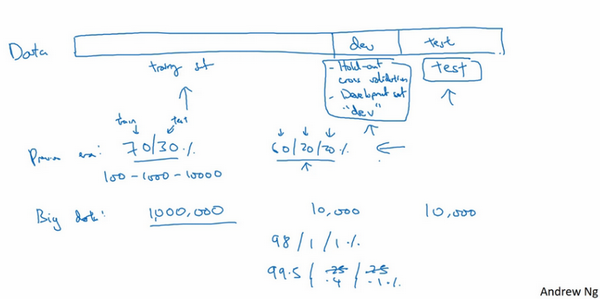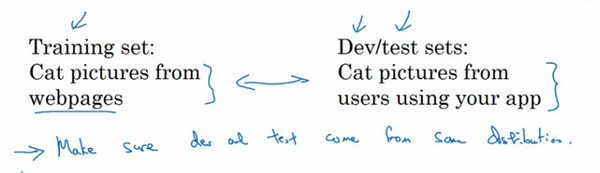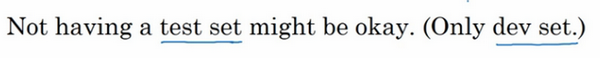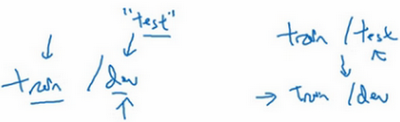### 1.2 偏差，方差（Bias /Variance）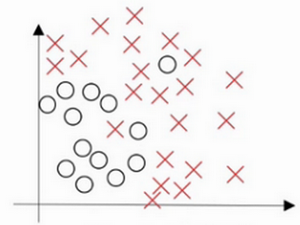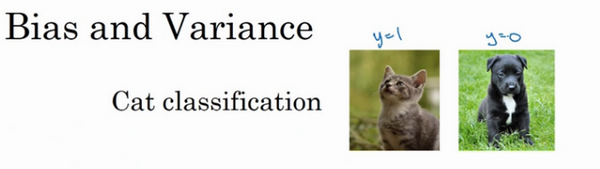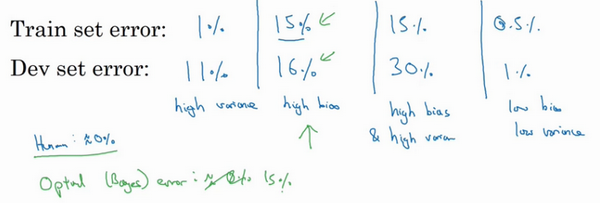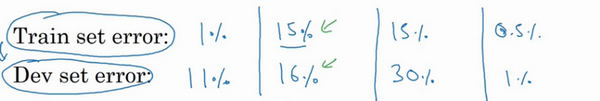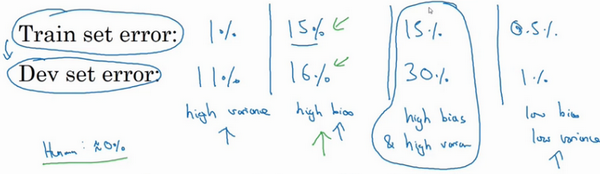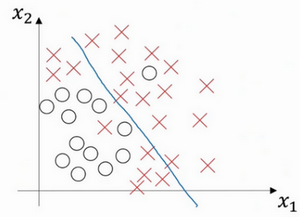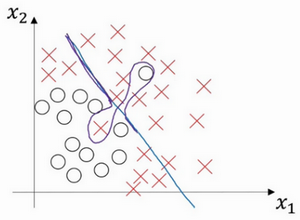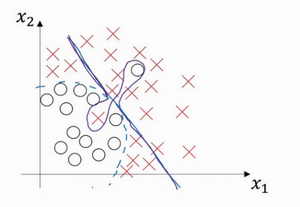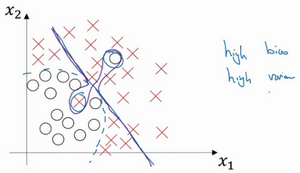### 1.3 机器学习基础（Basic Recipe for Machine Learning）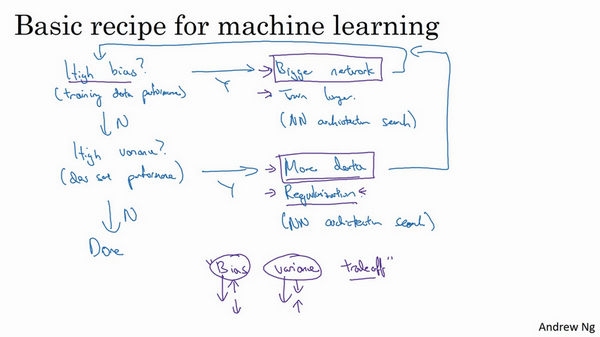### 1.4 正则化（Regularization）

$\frac{\lambda}{2m}$乘以$w$范数的平方，$w$欧几里德范数的平方等于$w_{j}$$j$ 值从1到$n_{x}$）平方的和，也可表示为$w^{T}w$，也就是向量参数$w$ 的欧几里德范数（2范数）的平方，此方法称为$L2$正则化。因为这里用了欧几里德法线，被称为向量参数$w$$L2$范数。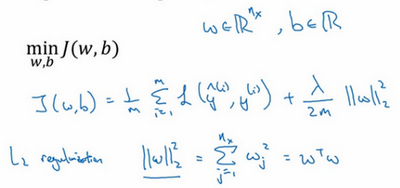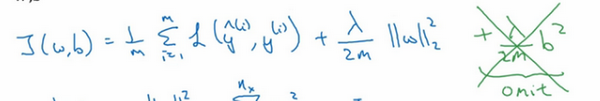$L2$正则化是最常见的正则化类型，你们可能听说过$L1$正则化，$L1$正则化，加的不是$L2$范数，而是正则项$\frac{\lambda}{m}$乘以$\sum_{j= 1}^{n_{x}}{|w|}$$\sum_{j =1}^{n_{x}}{|w|}$也被称为参数$w$向量的$L1$范数，无论分母是$m$还是$2m$，它都是一个比例常量。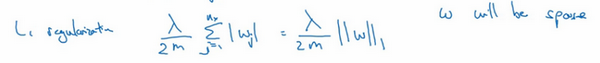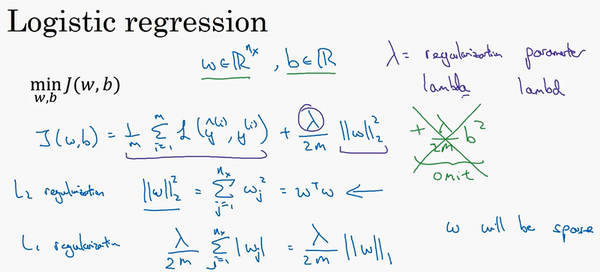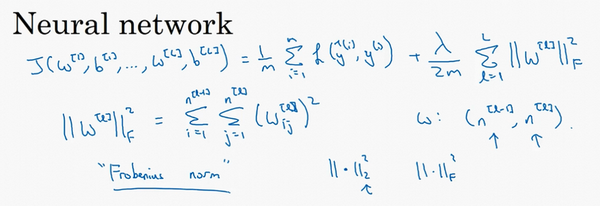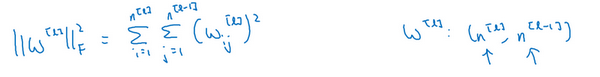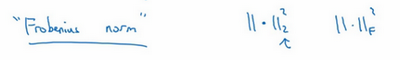backprop计算出$dW$的值，backprop会给出$J$对​$W$的偏导数，实际上是​$W^{[l]}$，把​$W^{[l]}$替换为​$W^{[l]}$减去学习率乘以​$dW$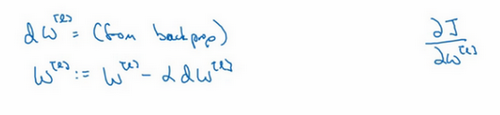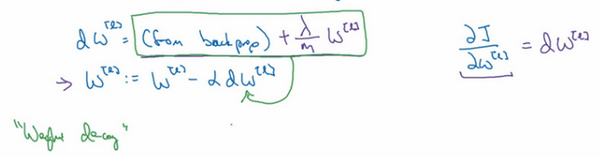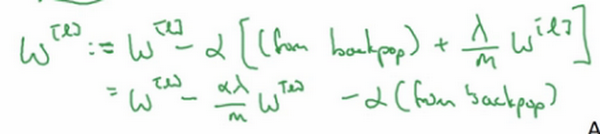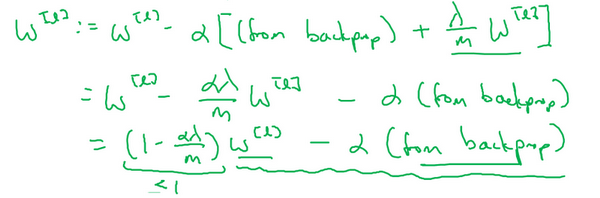### 1.5 为什么正则化有利于预防过拟合呢？（Why regularization reduces overfitting?）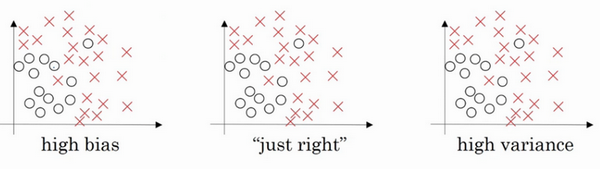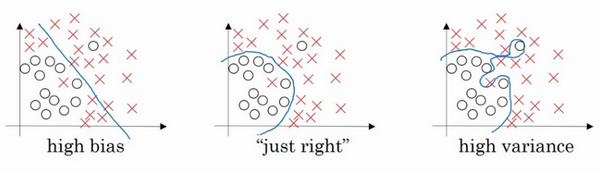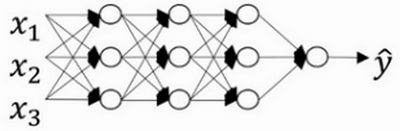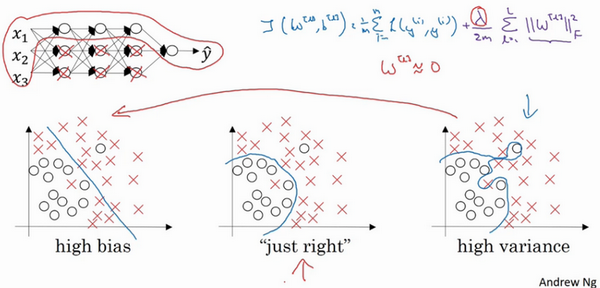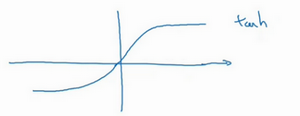$g(z)$表示$tanh(z)$,那么我们发现，只要$z$非常小，如果$z$只涉及少量参数，这里我们利用了双曲正切函数的线性状态，只要$z$可以扩展为这样的更大值或者更小值，激活函数开始变得非线性。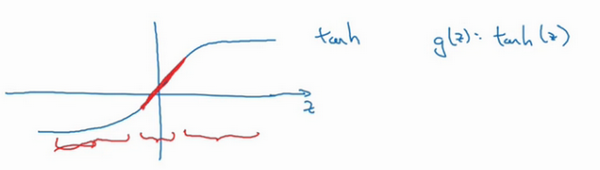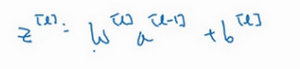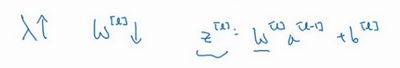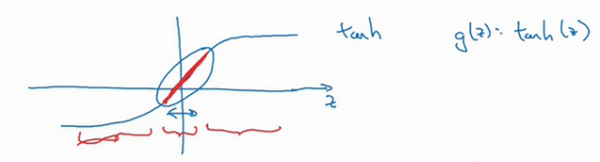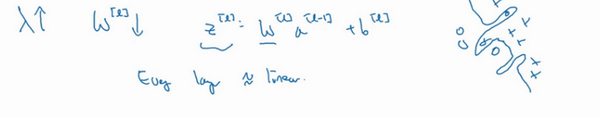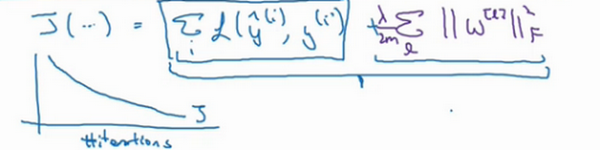### 1.6 dropout 正则化（Dropout Regularization）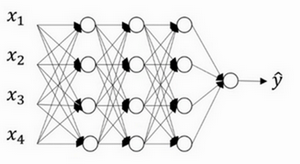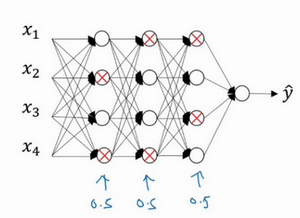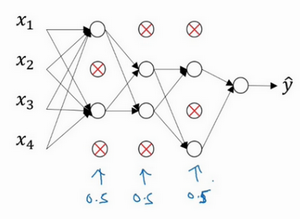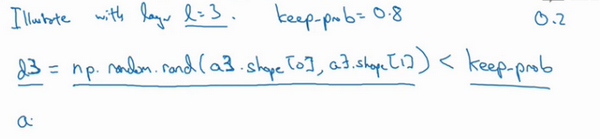d3 = np.random.rand(a3.shape,a3.shape)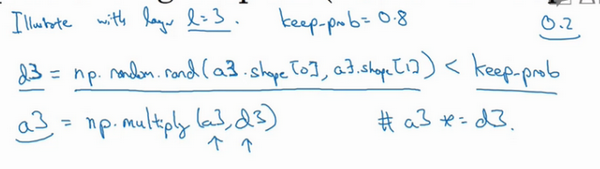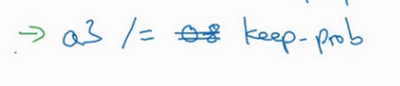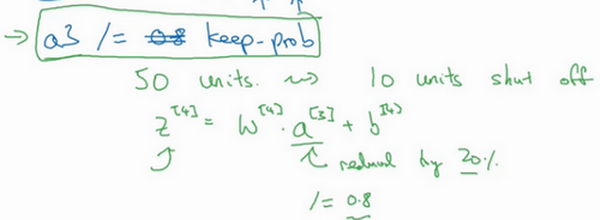$z^{} = w^{} a^{} + b^{}$

$a^{} = g^{}(z^{})$

$z^{} = \ w^{} a^{} + b^{}$

$a^{} = \ldots$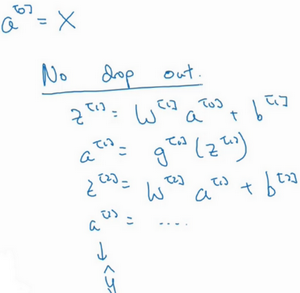Inverted dropout函数在除以keep-prob时可以记住上一步的操作，目的是确保即使在测试阶段不执行dropout来调整数值范围，激活函数的预期结果也不会发生变化，所以没必要在测试阶段额外添加尺度参数，这与训练阶段不同。

$l=keep-prob$

### 1.7 理解 dropout（Understanding Dropout）

Dropout可以随机删除网络中的神经单元，他为什么可以通过正则化发挥如此大的作用呢？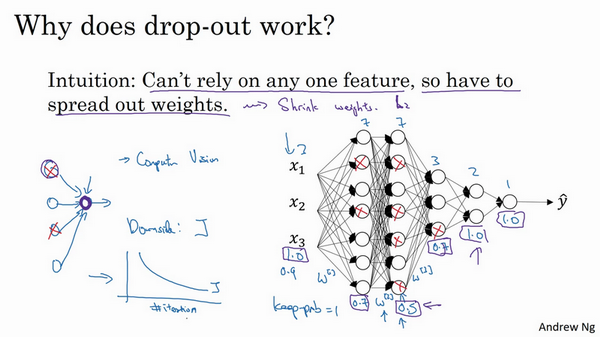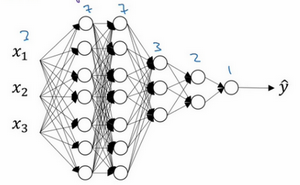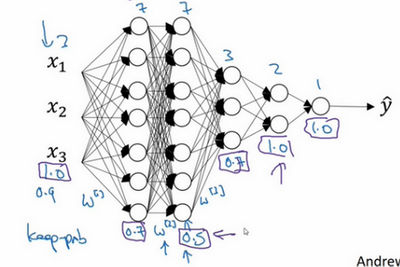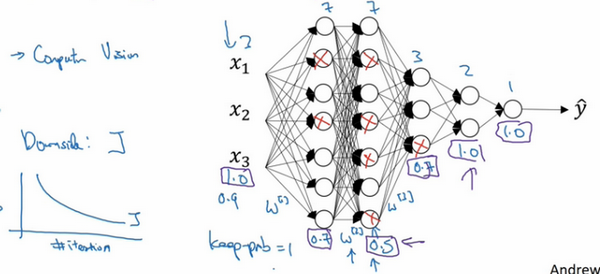dropout一大缺点就是代价函数$J$不再被明确定义，每次迭代，都会随机移除一些节点，如果再三检查梯度下降的性能，实际上是很难进行复查的。定义明确的代价函数$J$每次迭代后都会下降，因为我们所优化的代价函数$J$实际上并没有明确定义，或者说在某种程度上很难计算，所以我们失去了调试工具来绘制这样的图片。我通常会关闭dropout函数，将keep-prob的值设为1，运行代码，确保J函数单调递减。然后打开dropout函数，希望在dropout过程中，代码并未引入bug。我觉得你也可以尝试其它方法，虽然我们并没有关于这些方法性能的数据统计，但你可以把它们与dropout方法一起使用。

### 1.8 其他正则化方法（Other regularization methods）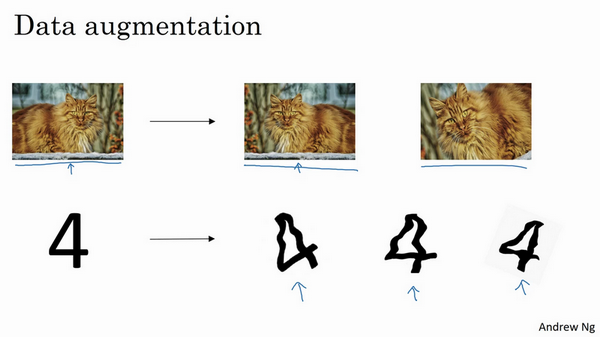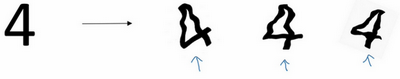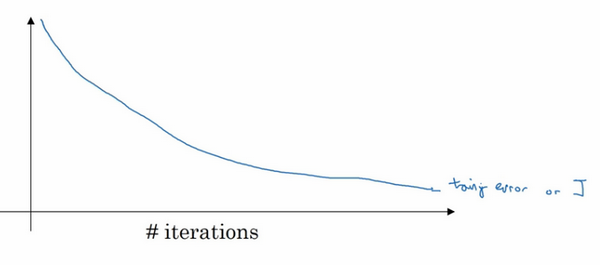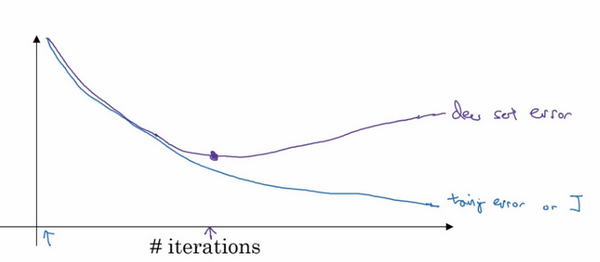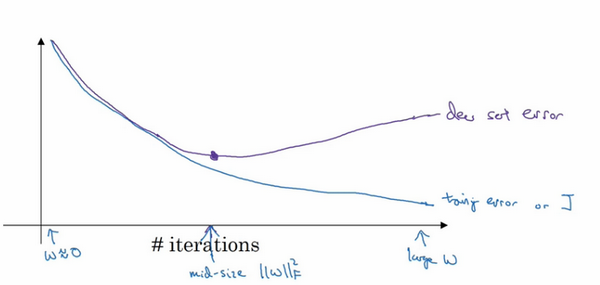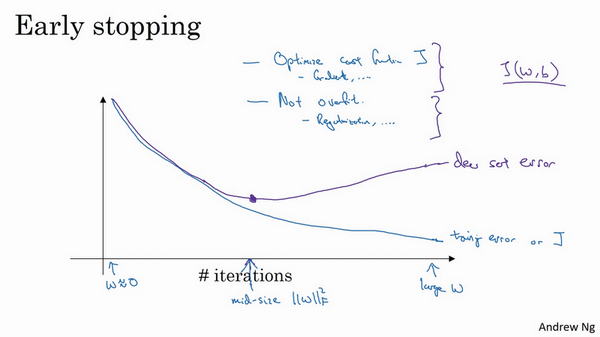Early stopping的优点是，只运行一次梯度下降，你可以找出$w$的较小值，中间值和较大值，而无需尝试$L2$正则化超级参数$\lambda$的很多值。

### 1.9 归一化输入（Normalizing inputs）

1. 零均值

2. 归一化方差；

我们希望无论是训练集和测试集都是通过相同的$μ$$σ^2$定义的数据转换，这两个是由训练集得出来的。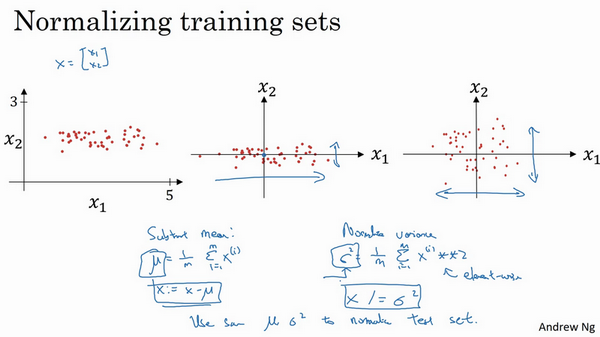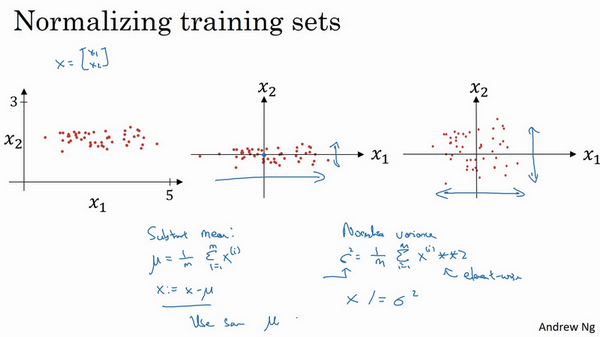$x_{1}$$x_{2}$的方差都等于1。提示一下，如果你用它来调整训练数据，那么用相同的 $μ$$\sigma^{2}$来归一化测试集。尤其是，你不希望训练集和测试集的归一化有所不同，不论$μ$的值是什么，也不论$\sigma^{2}$的值是什么，这两个公式中都会用到它们。所以你要用同样的方法调整测试集，而不是在训练集和测试集上分别预估$μ$$\sigma^{2}$。因为我们希望不论是训练数据还是测试数据，都是通过相同μ和$\sigma^{2}$定义的相同数据转换，其中$μ$$\sigma^{2}$是由训练集数据计算得来的。

$J(w,b)=\frac{1}{m}\sum\limits_{i=1}^{m}{L({{{\hat{y}}}^{(i)}},{{y}^{(i)}})}$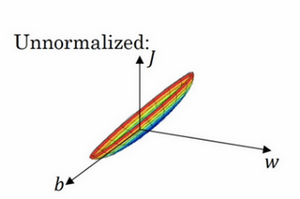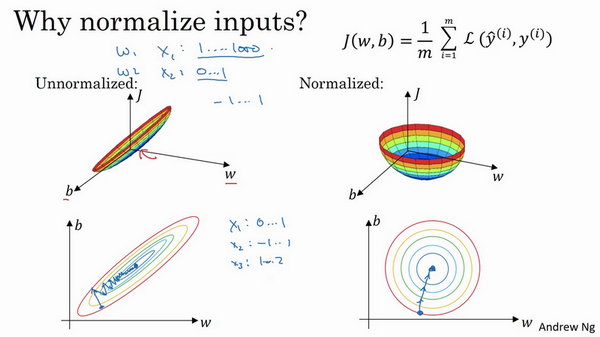### 1.10 梯度消失/梯度爆炸（Vanishing / Exploding gradients）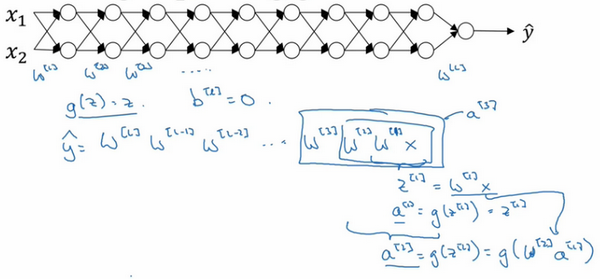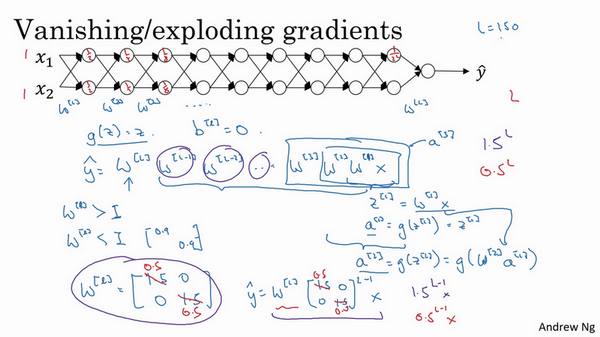### 1.11 神经网络的权重初始化（Weight Initialization for Deep NetworksVanishing / Exploding gradients）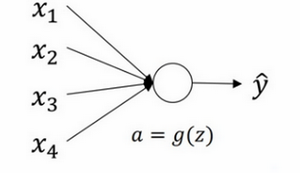$z = w_{1}x_{1} + w_{2}x_{2} + \ldots +w_{n}x_{n}$$b=0$，暂时忽略$b$，为了预防$z$值过大或过小，你可以看到$n$越大，你希望$w_{i}$越小，因为$z$$w_{i}x_{i}$的和，如果你把很多此类项相加，希望每项值更小，最合理的方法就是设置$w_{i}=\frac{1}{n}$$n$表示神经元的输入特征数量，实际上，你要做的就是设置某层权重矩阵$w^{[l]} = np.random.randn( \text{shape})*\text{np.}\text{sqrt}(\frac{1}{n^{[l-1]}})$$n^{[l - 1]}$就是我喂给第$l$层神经单元的数量（即第$l-1$层神经元数量）。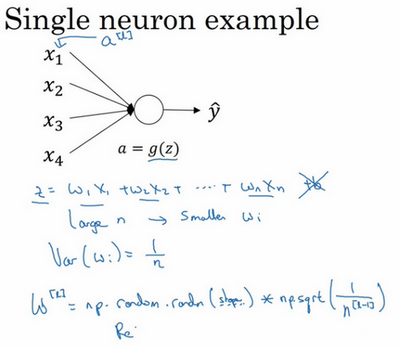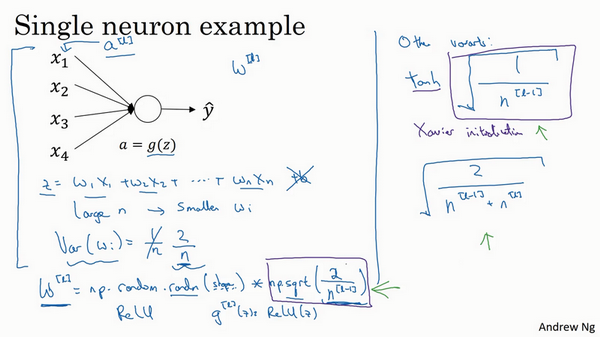### 1.12 梯度的数值逼近（Numerical approximation of gradients）